# JS中的算法与数据结构之二叉查找树（Binary Sort Tree）实例详解

## 二叉树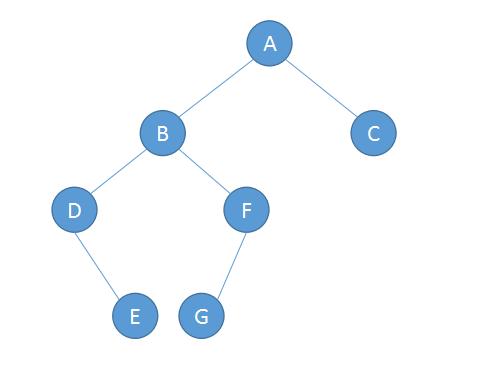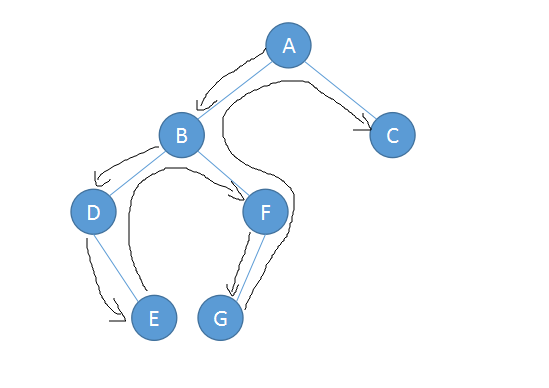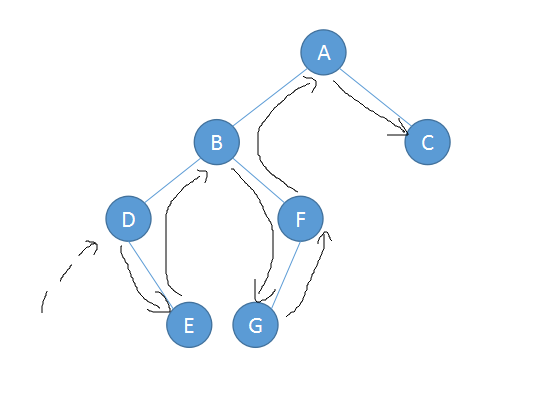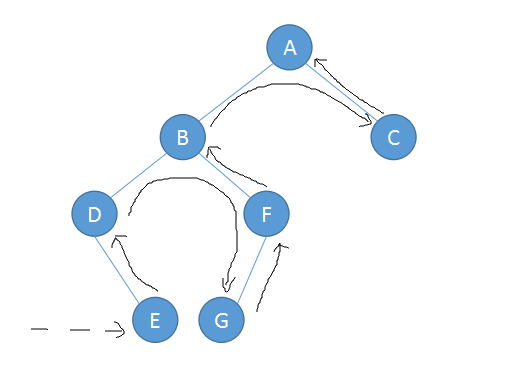## 二叉查找树的实现

```//节点定义

function Node (data , left , right) {
this.data = data;    // 数据
this.left = left;    // 左节点
this.right = right;   // 右节点
this.show = show;    // 显示节点数据
}

function show(){
return this.data;
}
```

Node对象既保存了数据，也保存了它的左节点和右节点的链接，其中 show 方法用来显示当前保存在节点中数据。

```//二叉查找树（BST）的类

function BST(){
this.root = null;      // 根节点
this.insert = insert;    // 插入节点
this.preOrder = preOrder;  // 先序遍历
this.inOrder = inOrder;   // 中序遍历
this.postOrder = postOrder; // 后序遍历
this.find = find;      // 查找节点
this.getMin = getMin;    // 查找最小值
this.getMax = getMax;    // 查找最大值
this.remove = remove;    // 删除节点
}
```

insert：向树中添加新节点

1. 设值当前节点为根节点
2. 如果待插入节点保存的数据小于当前节点，则新节点为原节点的左节点，反之，执行第4步
3. 如果当前节点的左节点为null，就将新节点放到这个位置，退出循环；反之，继续执行下一次循环
4. 设置新节点为原节点的右节点
5. 如果当前节点的右节点为null，就将新节点放到这个位置，退出循环；反之，继续执行下一次循环

```//插入新节点

function insert(data) {
var n = new Node( data , null , null );
if( this.root == null ){
this.root = n;
}else{
var current = this.root;
var parent;
while( true ){
parent = current;
if( data < current.data ){
current = current.left;
if( current == null ){
parent.left = n ;
break;
}
}else{
current = current.right;
if( current == null ){
parent.right = n;
break;
}
}
}
}
}
```

inOrder：中序遍历

``` // 中序遍历

function inOrder (node) {
if( !(node == null )){
inOrder( node.left );
console.debug( node.show() + ' ');
inOrder( node.right );
}
}
```

```var nums = new BST();
//插入数据
nums.insert(23);
nums.insert(45);
nums.insert(16);
nums.insert(37);
nums.insert(3);
nums.insert(99);
nums.insert(22);
```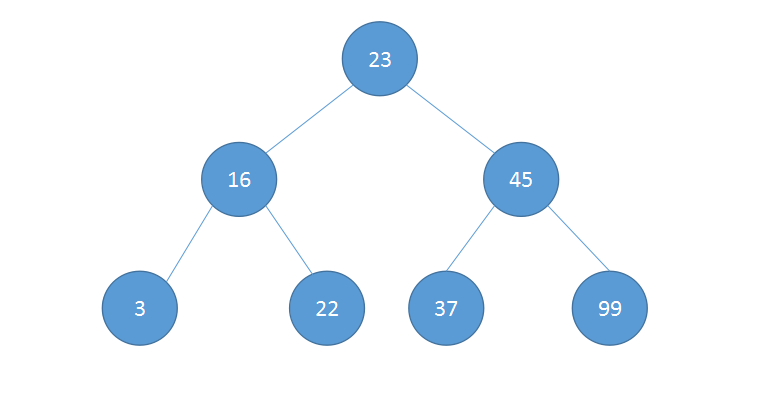BST

```//中序遍历
console.log("Inorder traversal: ");
inOrder(nums.root);

// Inorder traversal:
// 3 16 22 23 37 45 99
```

preOrder：先序遍历

```//先序遍历

function preOrder( node ) {
if( !(node == null )){
console.log( node.show() + ' ');
preOrder( node.left );
preOrder( node.right );
}
}
```

```// 先序遍历

console.log("Preorder traversal: ");
preOrder(nums.root);

// Preorder traversal:
// 23 16 3 22 45 37 99
```

postOrder：后序遍历

```//后序遍历

function postOrder ( node ) {
if( !(node == null ) ){
postOrder( node.left );
postOrder( node.right );
console.log( node.show() + ' ');
}
}
```

```// 后序遍历

console.log("Postorder traversal: ");
postOrder(nums.root);

// Postorder traversal:
// 3 22 16 37 99 45 23
```

## 二叉查找树的查找运算

1. 查找给定值
2. 查找最大值
3. 查找最小值

getMin：查找最小值

```//查找最小值

function getMin( ) {
var current = this.root;
while ( !( current.left == null ) ){
current = current.left;
}
return current.show();
}
```

getMax：查找最大值

```//查找最大值

function getMax () {
var current = this.root;
while ( !( current.right == null ) ) {
current = current.right;
}
return current.show();
}
```

```// 最小值
console.log('min:' + nums.getMin() );    // min : 3

//最大值
console.log('max:' + nums.getMax() );    // max : 99
```

```//查找给定值

function find ( data ) {
var current = this.root;
while ( current != null ){
if( current.data == data ){
return current;
}else if( current.data < data ){
current = current.right;
}else{
current = current.left;
}
}
return null;
}
```

```//查找不存在的值
console.log('find:' + nums.find(66));    // find : null

//查找存在的值
console.log('find:' + nums.find(99) );   // find : [object Object]
```

## 二叉查找树的删除运算

1. 首先判断当前节点是否包含待删除的数据，如果包含，则删除该节点；如果不包含，则比较当前节点上的数据和待删除树的的大小关系。如果待删除的数据小于当前节点的数据，则移至当前节点的左子节点继续比较；如果大于，则移至当前节点的右子节点继续比较。
2. 如果待删除节点是叶子节点（没有子节点），那么只需要将从父节点指向它的链接指向变为null；
3. 如果待删除节点含有一个子节点，那么原本指向它的节点需要做调整，使其指向它的子节点；
4. 最后，如果待删除节点包含两个子节点，可以选择查找待删除节点左子树上的最大值或者查找其右子树上的最小值，这里我们选择后一种。

```//删除操作

function remove( data ) {
removeNode( this.root , data);
}

//查找最小值

function getSmallest(node) {
if (node.left == null) {
return node;
}
else {
return getSmallest(node.left);
}
}

//删除节点
function removeNode( node , data ) {
if( node == null ) {
return null;
}
if(data == node.data) {
// 没有子节点的节点
if(node.left == null && node.right == null) {
return null;
}
// 没有左子节点的节点
if(node.left == null) {
return node.right;
}
// 没有右子节点的节点
if(node.right == null) {
return node.left;
}
// 有2个子节点的节点
var tempNode = getSmallest(node.right);
node.data = tempNode.data;
node.right = removeNode(node.right,tempNode.data);
return node;

}else if(data < node.data) {
node.left = removeNode( node.left,data);
return node;
}else {
node.right = removeNode( node.right,data);
return node;
}
}
```

```//删除根节点
nums.remove(23);

inOrder(nums.root);

// 3 16 22 37 45 99
```

JavaScript/Ajax教程-JS中的算法与数据结构之二叉查找树（Binary Sort Tree）实例详解